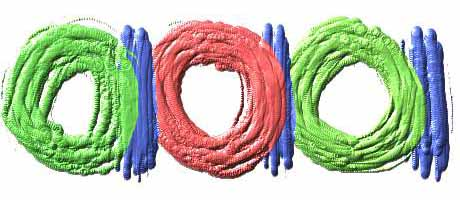Copyright © Dean Johnson 1999 (johnson@wmich.edu)

## Quiz#3: Binary Numbers

 The following quiz deals with the subject of the Binary Number System in the lecture notes. The questions test your ability to make conversions from one number base to another. Go over the appropriate reading assignments for this material, as specified by the course syllabus, and answer the following questions. The first ECE 250 exam will have questions appearing very similar to the ones given here. (Go back to homepage.) Converting (153)10 to base 8 yields which of the following results? Converting (153)8 to base 10 yields which of the following results? Converting (1010111)2 to base 8 yields which of the following results? The two's complement of the number (01010)2 is: Converting (11011.01)2 to base 8 yields which of the following results? Converting (.375)10 to base 2 yields which of the following results? Converting (169)10 to base 16 yields which of the following results? 10111 is the two's complement representation of: Converting (0111011.100)2 to base 16 yields which of the following results? 00111 is the two's complement representation of: Converting (187)10 to base 8 yields which of the following results? 10100 is the two's complement representation of: